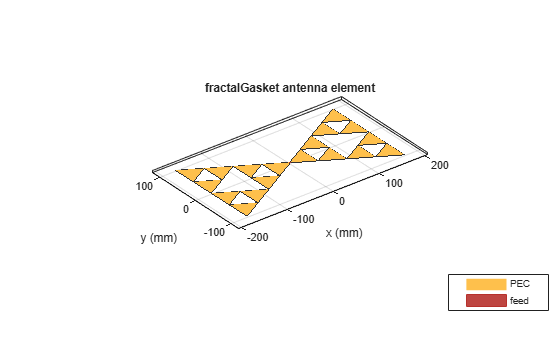Main Content

# fractalGasket

Create Sierpinski's Gasket fractal antenna on X-Y plane

## Description

The `fractalGasket` object creates an equilateral triangle-shaped Sierpinski's Gasket fractal antenna. These fractals are used in building communications systems, wireless networks, universal tactic communications systems, mobile devices, telematics, and radio frequency identification (RFID) antennas.

A fractal antenna uses a self-similar design to maximize the length or increase the perimeter of a material that transmits or receives electromagnetic radiation within a given volume or area. The main advantage of fractal antennas is that they are compact, which is important requirement for small and complex circuits. Fractal antennas also have more input impedance or resistance due to increased length or perimeter.

All fractal antennas are printed structures that are etched on a dielectric substrate.## Creation

### Syntax

``ant = fractalGasket``
``ant = fractalGasket(Name,Value)``

### Description

````ant = fractalGasket` creates an equilateral triangle-shaped Sierpinski’s gasket fractal antenna. The default planar fractal antenna is in the shape of a bowtie which is center-fed. The antenna resonates at a frequency of 1.3 GHz.```

example

````ant = fractalGasket(Name,Value)` sets properties using one or more name-value pairs. For example, `ant = fractalGasket('NumIterations',4)` creates a Sierpinski's Gasket with four iterations.```

## Properties

expand all

Number of iterations of the fractal antenna, specified as a scalar integer.

Example: `'NumIterations',2`

Example: `ant.NumIterations = 2`

Data Types: `double`

Lengths for three sides of the triangle, specified as a scalar in meters or a two- or three-element vector in meters.

• Scalar – The triangle is equilateral.

• Two-element vector – The first value specifies the base of the triangle along the X-axis. The second value specifies the other two sides of the triangle. The triangle is isosceles.

• Three-element vector – The first value specifies the base of the triangle along the X-axis. The remaining two values specify the other two sides of the triangle. The triangle is scalene.

Example: `'Side',[0.5000,1.000]`

Example: `ant.Side = [0.5000,1.000]`

Data Types: `double`

Width at the neck of the fractal antenna where the feed is located, specified as a positive scalar integer in meters.

Example: `'NeckWidth',0.0050`

Example: `ant.NeckWidth = 0.0050`

Data Types: `double`

Type of the metal used as a conductor, specified as a metal material object. You can choose any metal from the `MetalCatalog` or specify a metal of your choice. For more information, see `metal`. For more information on metal conductor meshing, see Meshing.

Example: ```m = metal('Copper'); 'Conductor',m```

Example: ```m = metal('Copper'); ant.Conductor = m```

Lumped elements added to the antenna feed, specified as a lumped element object handle. You can add a load anywhere on the surface of the antenna. By default, the load is at the origin. For more information, see `lumpedElement`.

Example: `'Load',lumpedelement`. `lumpedelement` is the object handle for the load created using `lumpedElement`.

Example: ```ant.Load = lumpedElement('Impedance',75)```

Tilt angle of the antenna, specified as a scalar or vector with each element unit in degrees. For more information, see Rotate Antennas and Arrays.

Example: `'Tilt',90`

Example: `ant.Tilt = 90`

Example: `'Tilt',[90 90]`,`'TiltAxis',[0 1 0;0 1 1]` tilts the antenna at 90 degrees about the two axes defined by the vectors.

Note

The `wireStack` antenna object only accepts the dot method to change its properties.

Data Types: `double`

Tilt axis of the antenna, specified as:

• Three-element vector of Cartesian coordinates in meters. In this case, each coordinate in the vector starts at the origin and lies along the specified points on the X-, Y-, and Z-axes.

• Two points in space, each specified as three-element vectors of Cartesian coordinates. In this case, the antenna rotates around the line joining the two points in space.

• A string input describing simple rotations around one of the principal axes, 'X', 'Y', or 'Z'.

For more information, see Rotate Antennas and Arrays.

Example: `'TiltAxis',[0 1 0]`

Example: `'TiltAxis',[0 0 0;0 1 0]`

Example: `ant.TiltAxis = 'Z'`

Note

The `wireStack` antenna object only accepts the dot method to change its properties.

Data Types: `double`

## Object Functions

 `show` Display antenna or array structure; display shape as filled patch `axialRatio` Axial ratio of antenna `beamwidth` Beamwidth of antenna `charge` Charge distribution on metal or dielectric antenna or array surface `current` Current distribution on metal or dielectric antenna or array surface `design` Design prototype antenna or arrays for resonance at specified frequency `efficiency` Radiation efficiency of antenna `EHfields` Electric and magnetic fields of antennas; Embedded electric and magnetic fields of antenna element in arrays `impedance` Input impedance of antenna; scan impedance of array `mesh` Mesh properties of metal or dielectric antenna or array structure `meshconfig` Change mesh mode of antenna structure `optimize` Optimize antenna or array using SADEA optimizer `pattern` Radiation pattern and phase of antenna or array; Embedded pattern of antenna element in array `patternAzimuth` Azimuth pattern of antenna or array `patternElevation` Elevation pattern of antenna or array `returnLoss` Return loss of antenna; scan return loss of array `sparameters` S-parameter object `vswr` Voltage standing wave ratio of antenna

## Examples

collapse all

Create and view a default fractal Sierpinski's Gasket.

`ant = fractalGasket`
```ant = fractalGasket with properties: NumIterations: 2 Side: 0.2000 NeckWidth: 0.0020 Conductor: [1x1 metal] Tilt: 0 TiltAxis: [1 0 0] Load: [1x1 lumpedElement] ```
`show(ant)`## See Also

### Topics

Introduced in R2018b

## Support

#### Hybrid Beamforming for Massive MIMO Phased Array Systems

Download the white paper